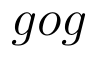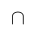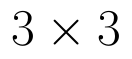Select Page

# CBSE MCQ in English Solutions for Maths 12 Science Relations and Functions

CBSE MCQ in English Solutions for Maths 12 Science Relations and Functions to enable students to get Solutions in a narrative video format for the specific question.

Expert Teacher provides CBSE MCQ Solutions for Maths 12 Science Relations and Functions through Video Solutions in English language. This video solution will be useful for students to understand how to write an answer in exam in order to score more marks. This teacher uses a narrative style for a question from Relations and Functions not only to explain the proper method of answering question, but deriving right answer too.

Please find the question below and view the Solution in a narrative video format.

Question:

Solution Video in English:

You can select video Solutions from other languages also. Please check Solutions in ( Hindi )

## Similar Questions from CBSE, 12th Science, Maths, Relations and Functions

Question 1 : Functionsare defined respectively, by, find(View Answer Video)

Question 2 : Number of binary sets on the setis, (View Answer Video)

Question 3 : The law a + b = b + a is called ______. (View Answer Video)

Question 4 : Functionsare defined respectively, by, find(View Answer Video)

Question 5 : Let A = {1, 2, 3, 4} and let R = {(2, 2), (3, 3), (4, 4), (1, 2)} be a relation on A. Then, R is, (View Answer Video)

### Integrals

Question 1 : Evaluate :(View Answer Video)

Question 2 : Evaluate the following :(View Answer Video)

Question 3 : Find the integral of the function. (View Answer Video)

Question 4 :(View Answer Video)

Question 5 : Given. Write f(x) satisfying the above.  (View Answer Video)

### Probability

Question 1 : Compute P(A/B) if P(B) =0.5 and P(AB)=0.32. (View Answer Video)

Question 2 : A bag contains 4 red and 4 black balls, another bag contains 2 red and 6 black balls. One of the two bags is selected at random and two balls are drawn at random (without replacement) from the bag which are both found to be red. Find the probability that the balls are drawn from the first bag.    (View Answer Video)

Question 3 : There are three coins. First is a biased that comes up tails 60% of the times, second is also a biased coin that comes up heads 75% of the times and third is an unbiased coin. One of the three coins is chosen at random and tossed, it shows heads, what is the probability that it was the first coin?      (View Answer Video)

Question 4 : A bag X contains 4 white balls and 2 black balls, while another bag Y contains 3 white balls and 3 black balls. Two balls are drawn (without replacement) at random from one of the bags and were found to be one white and one black. Find the probability that the balls were drawn from bag Y.  (View Answer Video)

Question 5 :  Bag I contains 3 red and 4 black balls and Bag II contains 4 red and 5 black balls. Two balls are transferred at random from Bag I to Bag II and then a ball is drawn from Bag II.  The ball so drawn is found to be red in colour. Find the probability that the transferred balls were both black.   (View Answer Video)

### Determinant

Question 1 : Find the inverse of the matrix. (View Answer Video)

Question 2 : Let A be the non- singular square matrix of order, then |adj A| is equal to, (View Answer Video)

Question 4 : If A is a symmetric matrix, then? (View Answer Video)
Question 5 : Find the values of x, if. (View Answer Video)